# Fast Polynomial Multiplication based on the Discrete Fourier Transform

In the whole section we consider a commutative ring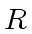with units. Let us consider two univariate polynomials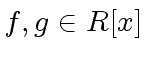of degree less than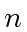. Then their product has degree less than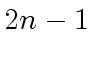. Assume that we are given a finite subset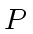ofwith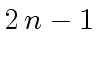elements such that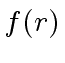and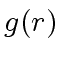are known for every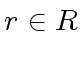. Then the values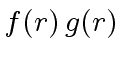define the polynomial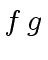completely. Indeed, by Lagrange interpolation we construct the polynomial(which has at mostcoefficients) from thevalues. Observe that the cost of definingby the valuesis, which is cheap!

However if we need information aboutsuch as its leading coefficient or its number of terms we need to compute the coefficients of. The cost of this construction is in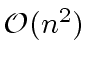.

The underlying idea of the fast polynomial multiplication based on the discrete Fourier transform is the following. Assume that there exists a subsetofwithelements such that

• evaluating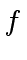and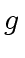at every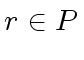can be done at nearly linear time cost (such as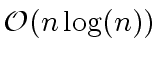),
• interpolatingforcan be done at nearly linear time cost.
Then the multiplication ofby, represented by their coefficients, can be done in.

Such setsdo not always exist. This depends on the ringand the degrees of the polynomialsand. However many finite fields possess such sets for small enough degrees ofand. For the arbitrary ring, it can be shown that the multiplication ofnad, represented by their coefficients, can be done in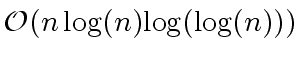.

Subsections
Marc Moreno Maza
2008-01-07